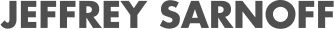# Calibration analysis of probabilistic models in Julia

07/30/2021, 12:30 PM1:00 PM UTC
Green

#### Abstract:

Calibrated probabilistic models ensure that predictions are consistent with empirically observed outcomes, and hence such models provide reliable uncertainty estimates for decision-making. This is particularly important in safety-critical applications. We present Julia packages for analyzing calibration of general probabilistic predictive models, beyond commonly studied classification models. Additionally, our framework allows to perform statistical hypothesis testing of calibration.

#### Description:

The Pluto notebook of this talk is available at https://talks.widmann.dev/2021/07/calibration/

The talk focuses on:

• introducing/explaining calibration of probabilistic models
• discussing/showing how users can apply the offered evaluation measures and hypothesis tests
• highlighting the relation to the Julia ecosystem, in particular to packages such as KernelFunctions and HypothesisTests and interfaces via pyjulia (Python) and JuliaCall (R)

Probabilistic predictive models, including Bayesian and non-Bayesian models, output probability distributions of targets that try to capture uncertainty inherent in prediction tasks and modeling. In particular in safety-critical applications, it is important for decision-making that the model predictions actually represent these uncertainties in a reliable, meaningful, and interpretable way.

A calibrated model provides such guarantees. Loosely speaking, if the same prediction would be obtained repeatedly, then it ensures that in the long run the empirical frequencies of observed outcomes are equal to this prediction. Note that usually it is not sufficient if a model is calibrated though: a constant model that always outputs the marginal distribution of targets, independently of the inputs, is calibrated but probably not very useful.

Commonly, calibration is analyzed for classification models, often also in a reduced binary setting that focuses on the most-confident predictions only. Recently, we published a framework for calibration analysis of general probabilistic predictive models, including but not limited to classification and regression models. We implemented the proposed methods for calibration analysis in different Julia packages such that users can incorporate them easily in their evaluation pipeline.

CalibrationErrors.jl contains estimators of different calibration measures such as the expected calibration error (ECE) and the squared kernel calibration error (SKCE). The estimators of the SKCE are consistent, and both unbiased and unbiased estimators exist. The package uses kernels from KernelFunctions.jl, and hence many standard kernels are supported automatically.

CalibrationTests.jl implements statistical hypothesis tests of calibration, so-called calibration tests. Most of these tests are based on the SKCE and can be applied to any probabilistic predictive model.

Finally, the package CalibrationErrorsDistributions.jl extends calibration analysis to models that output probability distributions from Distributions.jl. Currently, Gaussian distributions, Laplace distributions, and mixture models are supported.

To increase the adoption of these calibration evaluation techniques by the statistics and machine learning communities, we also published interfaces to the Julia packages in Python and R.

### References

Widmann, D., Lindsten, F., & Zachariah, D. (2019). Calibration tests in multi-class classification: A unifying framework. In Advances in Neural Information Processing Systems 32 (NeurIPS 2019) (pp. 12257–12267).

Widmann, D., Lindsten, F., & Zachariah, D. (2021). Calibration tests beyond classification. International Conference on Learning Representations (ICLR) 2021.